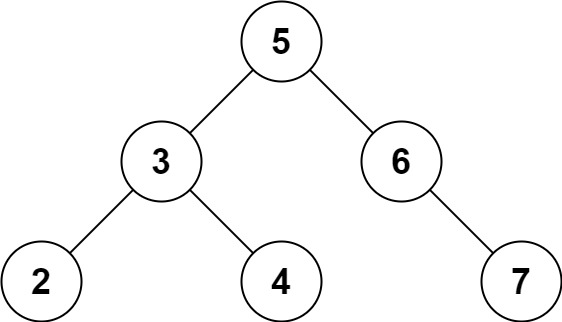| English | 简体中文 |

# 653. Two Sum IV - Input is a BST

## Description

Given the root of a Binary Search Tree and a target number k, return true if there exist two elements in the BST such that their sum is equal to the given target.

Example 1:Input: root = [5,3,6,2,4,null,7], k = 9
Output: true


Example 2:Input: root = [5,3,6,2,4,null,7], k = 28
Output: false


Constraints:

• The number of nodes in the tree is in the range [1, 104].
• -104 <= Node.val <= 104
• root is guaranteed to be a valid binary search tree.
• -105 <= k <= 105